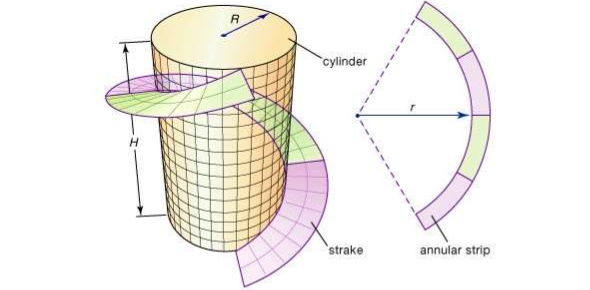# How Much Do You About Differential Geometry?

10 Questions | Total Attempts: 344SettingsDifferential geometry is a mathematical discipline that uses the techniques of differential calculus, integral calculus, linear algebra and multilinear algebra to study problems in geometry. The theory of plains and spaces curves and surfaces in the three-dimensional Euclidean space formed the basis for development of differential geometry during the 18th century and the 19th century.

• 1.
Differential geometry is a discipline of ......?
• A.

Mathematics

• B.

Music

• C.

Language

• D.

Philosophy

• 2.
Since what century has differential geometry has grown into a field concerned more generally with the geometric structures on differentiable manifold?
• A.

19th century

• B.

20th century

• C.

18th century

• D.

17th century

• 3.
The formal study of the nature of curves and surfaces became a field of study in its own right, with Monge's paper in ....?
• A.

1850

• B.

1888

• C.

1795

• D.

1997

• 4.
Differential geometry has how many branches?
• A.

9

• B.

8

• C.

4

• D.

7

• 5.
Which branch of geometry has the Finsler manifold as the main object of study?
• A.

Riemannian geometry

• B.

Finsler geometry

• C.

Simplectic geometry

• D.

Contact geometry

• 6.
Differential geometry is a topic under .....?
• A.

Geometry

• B.

Algebra

• C.

Vector spaces

• D.

Lattices

• 7.
What is the study of the intrinsic geometry of boundaries of domains in complex manifolds?
• A.

CR geometry

• B.

Contact geometry

• C.

Symplectic geometry

• D.

Lie groups

• 8.
Which geometry generalizes Riemannian geometry to the case in which the metric tensor need not be positive-definite?
• A.

Pseudo-Riemannian geometry

• B.

Complex geometry

• C.

CR geometry

• D.

Finsler geometry

• 9.
Differential system belongs to ....?
• A.

History

• B.

Philosophy

• C.

Languages

• D.

Mathematics

• 10.
Differential geometry uses the following techniques except .....?
• A.

Vector spaces

• B.

Linear algebra

• C.

Integral calculus

• D.

Differential calculus

Related TopicsBack to top